RSA-129

There is one famous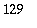digit number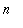in the literature, called RSA-, which was used as a challenge to anyone to break the message encrypted using thisand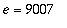.  That is, to break the message, one has to factor the number below into a product of two primesand, in order to findand then find the inverseof the number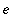modulo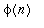. This number, called in the literature RSA_129  is:

 > RSA_129 := 114381625757888867669235779976146612010218296721242362562561842935706935245733897830597123563958705058989075147599290026879543541:

The exponentused to encrypt is:

 > e:=9007;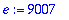Using the assignment of letters A=01, B=02, ... , Z=26 with a blank being 00, the RSA-encrypted message was:

 > C := 96869613754622061477140922254355882905759991124574319874695120930816298225145708356931476622883989628013391990551829945157815154:

The numberis known to be the product of a 64-digit primeand a 65-digit prime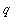. A \$100 prize was offered by the M.I.T group of Ronald L. Rivest, Adi Shamir, and Leonard Adleman for any one who could decrypt the message. That is, factor the above 129-digit (426-bit) number, then find, then find the inverseof.  To findwe need to be able to factorso that, The numberand the prize announcement can be found in Martin Gardner's delightful article in the August 1977 edition of Scientific American. Given the state of computer power in 1977, Rivest, Shamir, and Adleman estimated it would take some 20,000 years to factor it. The number was factored in March 1994 by Atkins et al. using the resources of 1600 computers (which included two fax machines) from the Internet. The factoring took about 4000 to 6000 MIPS years of computation over an eight-month period. It was factored using the quadratic sieve factoring method and, according to Lenstra, will perhaps be the last large number to be factored using the quadratic sieve since the general number field sieve is now more efficient for numbers of this size and larger.
The primes are:

 > p := 3490529510847650949147849619903898133417764638493387843990820577:

 > q := 32769132993266709549961988190834461413177642967992942539798288533:

 > RSA_129-p*q;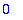> phi_RSA_129:=(p-1)*(q-1);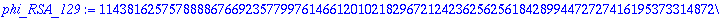> d:=e^(-1) mod phi_RSA_129;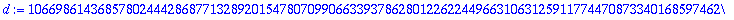> M:=C&^d mod RSA_129;Next we have to translate the numbers back to letters and blanks. We need the following functions to do this:

 > `crypt/alphabet` := `ABCDEFGHIJKLMNOPQRSTUVWXYZ `:

 > to_number := proc(st, string) local ll, nn, ss, ii;   ll := length(st); if ll = 0 then RETURN(0) fi; nn := 1; for ii to ll do ss := searchtext(substring(st, ii .. ii),                  `crypt/alphabet`); if ss=27 then ss:=00 fi; nn := 100*nn + ss od; nn - 10^(2*ll) end:

 > from_number := proc(nn, integer) local ss, mm, ll, pp, ii, ans; mm := nn; ll:=trunc((length(mm)+1)/2); ans := ``; for ii to ll do mm := mm/100; pp := 100*frac(mm); if pp=00 then pp:=27 fi; ss := substring(`crypt/alphabet`, pp..pp); ans := cat(ss, ans); mm := trunc(mm) od; ans end:

 >

Now we can see what the message M really says:

 > from_number(M);An ossifrage is an old word for an osprey which is a large hawk that feeds only on fish. To prove that the offer of \$100 actually came from the M.I.T group, the following signature was added:

 > SIG:=16717861150380844246015271389168398245436901032358311217835038446929062655448792237114490509578608655662496577974840004057020373:

SIG was encoded with the secretfound above. So if we raise SIG to the power 9007, it should tell us the signature.

 > SIG&^9007 mod RSA_129;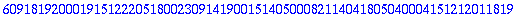> from_number(%);